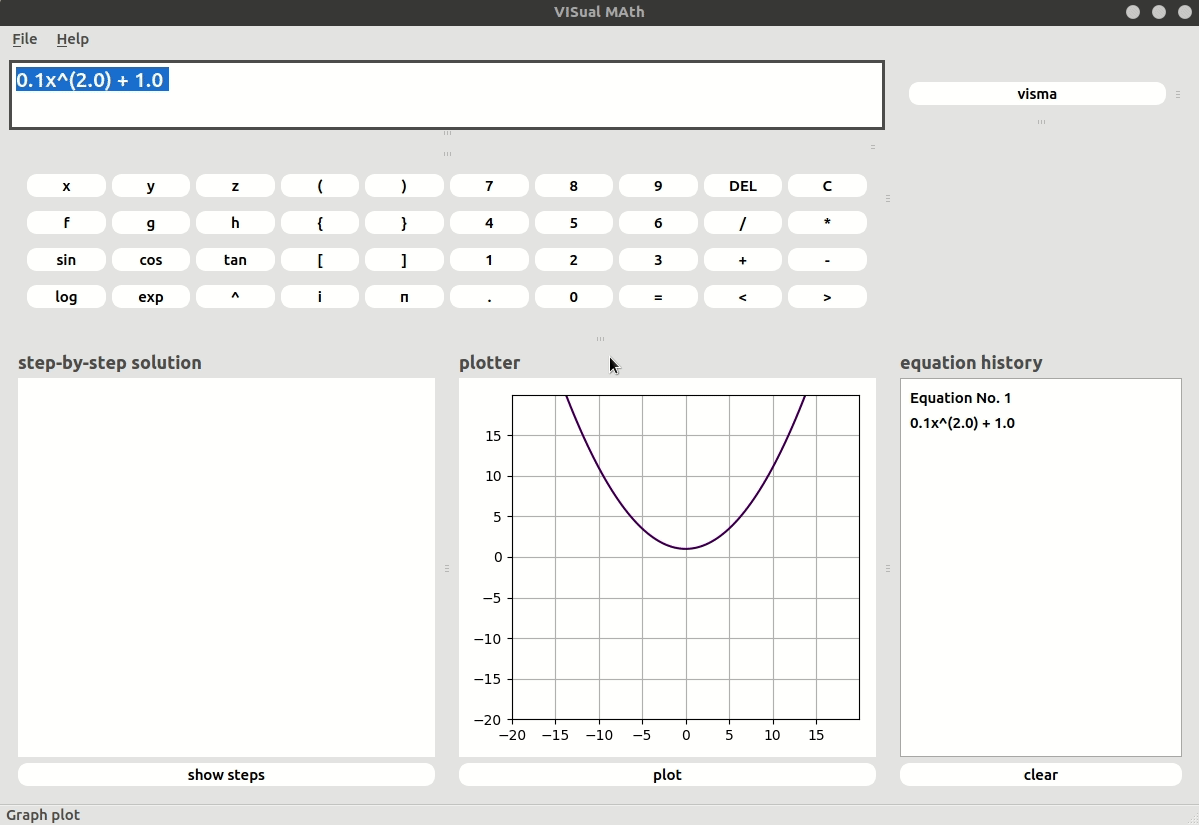# [GSoC’18|VisMa|Shantanu] Week #06-07: Finding the Unknown

This is GSoC’18 log#03. Here I will cover on what I have done in week#06-07. Link to the previous log.## Done so far…

I have implemented a simple solver which is capable of solving a given variable in terms of other variables and numbers. Below is a simple example of how the solver works.

```x^2*y - z = 1            # solving for x
x^2y - z - 1 = 0         # simplified and moved to LHS
x^2y = z + 1             # all functions not containing x moved to RHS
x^2 = (z + 1)/y          # inverse method is called
x = ((z + 1)/y)^0.5      # final result```

To make the solver some features like expression operations were required.
Also, support for nested expressions has been added. So when operating with expressions the operations will be worked out from inside-out.

While I was working on expressions, I missed a few trivial test cases. Since the number of functions/operations are increasing the number of test cases is increasing.

Hence I decided to automate testing using unit-test. Since this topic was new to me, I tried using various tests available to find which was best suited for visma. I started using doctest but later moved on to pytest for its number of features. Writing a test through pytest is as simple as:-

```from visma.utils.integers import gcd

def test_gcd(): # simple test for gcd
assert gcd() == 1
assert gcd([3, 6, 12, 24]) == 3
```

While working on these I learned a lot about unit-tests and code-coverage. I used coverage.py through pytest to measure code-coverage. It helps to know which part of the code is being neglected and helps find missing test cases.

I fiddled a little with the stylesheets in PyQt4 and made the GUI a little cleaner. Below is the solver in action.## What I will be doing next…

Since the solver for single equation has been implemented, the next thing will be to extend it to solve multiple equations at once. A variable substitution method will be written which will substitute a variable from one equation to the other equation.

Also for multi-variable linear equations, matrix modules will be created and Gauss-Jordan elimination will be used for solving these. The solver will also be modified to support inequality solving.

Since I have just initialized testing using pytest, there are many test cases left to be written. Tests for specifically each module will be created.